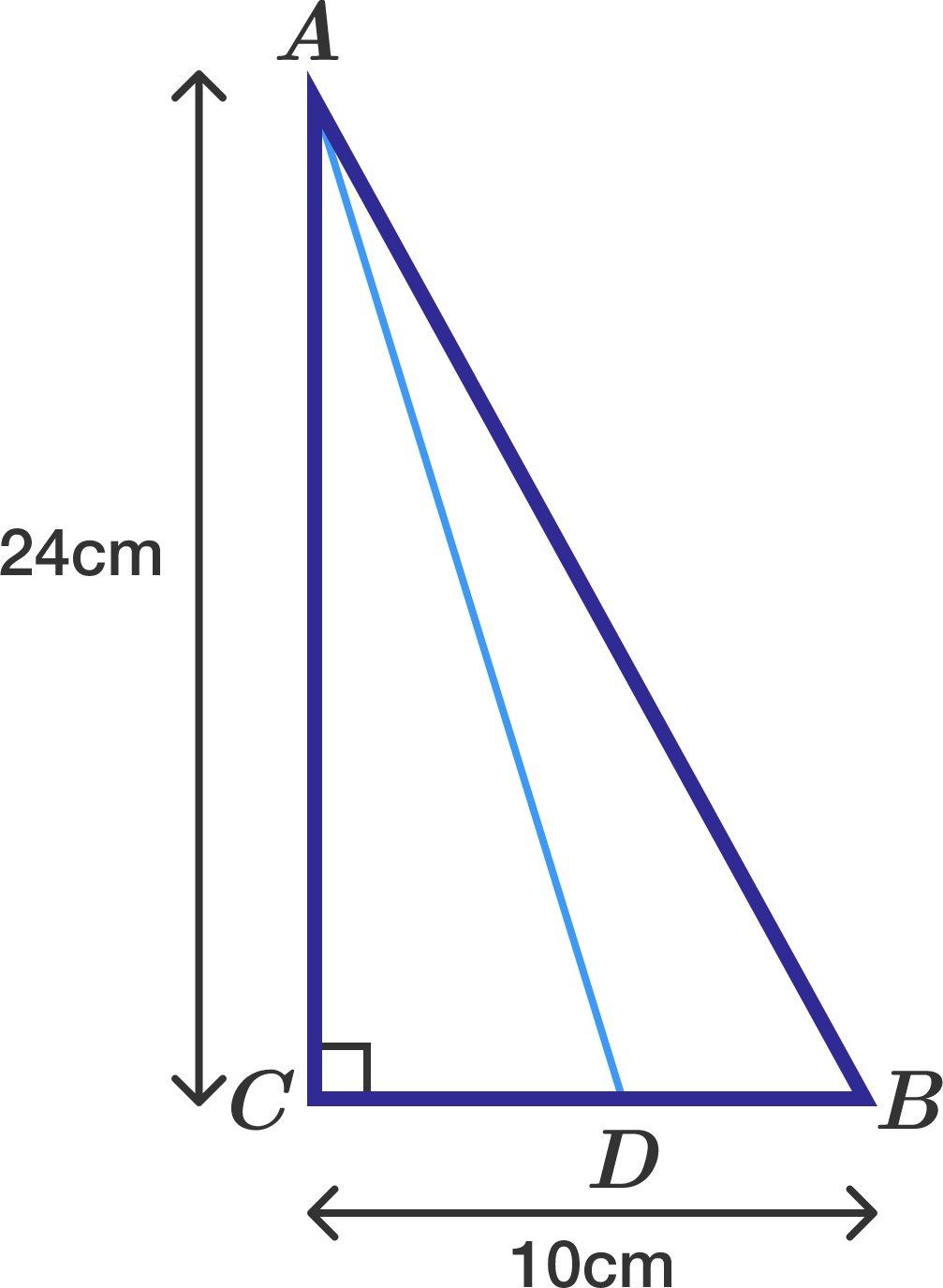# Reflection of C is on AB

Geometry Level 2A right angle triangle $ABC$ is such that $AC=24$cm, $CB=10$cm and $\angle ACB=90^{\circ}$. A point $D$ on $CB$ is such that when point $C$ is reflected upon line $AD$, the reflected point will be on line $AB$. The length of $CD=\frac{a}{b}$ where $a$ and $b$ are coprime integers. Find the value of $a+b$.

×